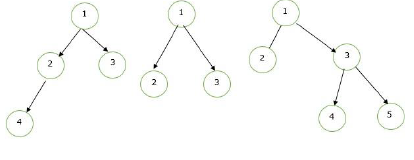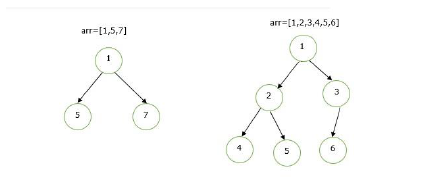# Python program to implement binary tree data structure

A tree is a data structure which consists of nodes. The nodes are connected by the edges. The top most node is called as the root and the bottom most nodes are called as the leaves. Leaves are the nodes that do not have any children.

## Binary Tree

A binary tree is a tree in which every node can consist a maximum of 2 children. That means, every node can either have 0 or 1 or 2 children but not more than that. Every node in a binary tree consists of three fields −

• Data

• Pointer to the left child

• Pointer to the right childComplete binary tree − A binary tree is said to be a complete binary tree if all the levels are complete filled except the last level and all the nodes should be as left as possible.

Strict/Proper binary tree − A binary tree is said to be a strict or proper binary tree if every node has either zero or two children.

Perfect binary tree − A binary tree is said to be a perfect binary tree if all the nodes have two childresn and all the leaf nodes are at the same level.

Balanced binary tree − A binary tree is said to be balanced if the difference between the height of the left sub tree and the height of right sub tree is at most one(0 or 1).

## Constructing binary tree from the given array### Example

If the root node is present at the ith index, then the left child will be present at the (2*i+1)th index and the right child will be present at the (2*i-1)th index. We will use this concept for constructing the binary tree from the array elements.

class TreeNode:
def __init__(self,data,left=None,right=None):
self.data=data
self.left=left
self.right=right
def insert_into_tree(root,arr,i,l):
if i<l:
print(arr[i])
temp=TreeNode(arr[i])
root=temp
root.left=insert_into_tree(root,arr,i*2+1,l)
root.right=insert_into_tree(root,arr,i*2+2,l)
return root
arr=[1,2,3,4,5]
i=0
l=len(arr)
root=TreeNode(arr)
insert_into_tree(root,arr,i,l)


### Output

1
2
4
5
3


Updated on: 24-Apr-2023

104 Views